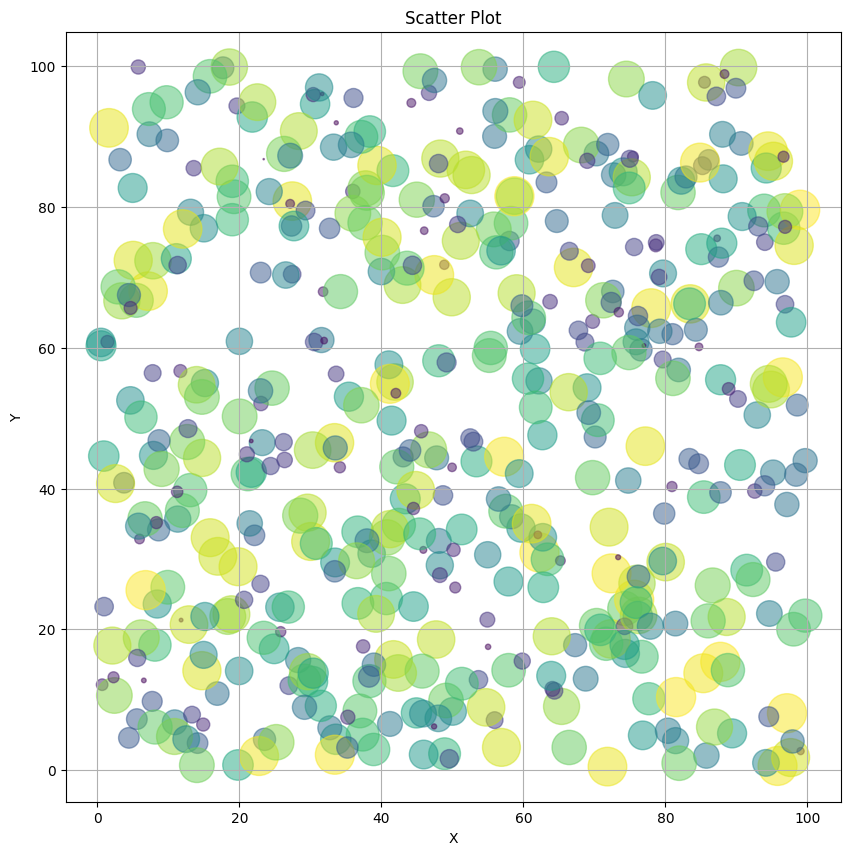This is a simple Julia 0.6 notebook. Below we'll show off the Julia PyPlot module.

In :
using PyPlot
ioff() # Interactive plotting OFF, necessary for inline plotting in IJulia

In :
x = 100 * rand(500)
y = 100 * rand(500)
areas = 800 * rand(500);

In :
#  Scatterplot
fig = figure("scatterplot",figsize=(10,10))
ax = axes()
scatter(x,y,s=areas,alpha=0.5, c=areas)

title("Scatter Plot")
xlabel("X")
ylabel("Y")
grid("on")
gcf();In [ ]: